# NCERT Solutions for Chapter 14 How Big? How Heavy? Class 5 MathsHow Big? How Heavy? Questions and Answers

 Chapter Name How Big? How Heavy? NCERT Solutions Class CBSE Class 5 Textbook Name Maths Related Readings NCERT Solutions for Class 5NCERT Solutions for Class 5 Maths Summary of How Big? How Heavy?

Page No 188:

Question 1: Guess the volume of each of these:

• A ball is nearly ____ marbles.
• An eraser is nearly ____ marbles.
• A lemon is nearly ____ marbles.
• A pencil is nearly ____ marbles.
• A potato is nearly ____ marbles.

• A ball is nearly 20 marbles.
• An eraser is nearly 2 marbles.
• A lemon is nearly 4 marbles.
• A pencil is nearly 2 marbles.
• A potato is nearly 10 marbles.

Disclaimer: The answer may vary from student to student. The answers provided here are for reference only.

Question 2: Try with different things like a matchbox, a stone, etc. and fill the table.

 Name of the thing Its volume (nearly how many marbles?)

 Name of the thing Its volume (nearly how many marbles?) A grape 1 marbles An egg 3 marbles 5 chalks 2 marbles 1 bowl of rice 10 marbles

Disclaimer: The answer may vary from student to student, based on his/her observation. It is highly recommended that the students prepare the answers on their own. The answers provided here are for reference only.

Page No 189:

Question 1: Use your measuring bottle to find out:
(a) What is the volume of 6 marbles? ____ mL.
(b) What is the volume of 16 one- rupee coins?____ mL.
Now solve these in your mind.
(c) The volume of 24 marbles is ____ mL.
(d) The volume of 32 one-rupee coins? ____ mL.
(e) Mollie puts some five-rupee coins in the measuring bottle. How many coins has she put in it
if 30 mL water is pushed up?____ if 60 mL water is pushed up?____ First guess and then use your measuring bottle to find out the volume in mL of some other things.

 Thing Its volume (mL)

Disclaimer: The answer may vary from student to student, based on his/her observation. It is highly recommended that the students prepare the answer on their own.

Page No 191:

Question 1:

1. A stage (platform) is made with 5 Math-Magic books. The volume of this stage is the same as ____ cm cubes.
2. Guess the volume of these things in cm cubes.
• A matchbox is about____ cm cubes.
• A geometry box is about____ cm cubes.
• An eraser is about____ cm cubes. How will you check your guess? Discuss.

1.  Volume of 1 Math-Magic book = 540 cm cubes. 5 Math-Magic books are used to make the stage. So, volume of the stage = Volume of 5 such Math-Magic books = 5 x 540 cm cubes = 2700 cm cubes

• A matchbox is about 13.5 cm cubes.
• A geometry box is about 157.5 cm cubes.
• An eraser is about 1.5 cm cubes.

We will multiply the length, breadth and height of the object to cross- check our guess.

Question 2: Tanu is making a stage with matchboxes. She first puts 14 matchboxes like this in the first layer. She makes 4 such layers and her stage looks like this.

• She used ____ matchboxes to make this stage.
• The volume of one matchbox is the same as 10 cm cubes. Then the volume of this stage is same as ____ cm cubes.
• If all these cubes are arranged in a line, how long will that line be?____ cm.
• Which has more volume --- your Math-Magic book or Tanu's platform? With your friends, collect many empty matchboxes of the same size. Measure the sides and write here.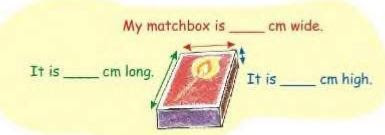• Use 56 matchboxes to make platforms of different heights. Fill this table.
 How high is it? How long is it? How wide is it? Platform 1 Platform 2 Platform 3

The volume of each platform is equal to ______matchboxes.

• Number of matchboxes in 1 layer = 14 Number of layers that make the stage = 4 Total number of matchboxes in 4 layers = 14 × 4 = 56

• Volume of 1 matchbox = 10 cm cubes A total of 56 matchboxes are used to make the stage. So, the volume of this stage = 56 x 10 cm cubes = 560 cm cubes.

• Now, let's assume length of matchbox is 4.5 cm. All the matchboxes are arranged in a line. Then the total length of the line made by all 56 matchboxes = 4.5 cm x 56 = 252 cm

• The volume of Math-Magic book is 540 cm cubes, whereas volume of the platform is 560 cm cubes. Thus, Tanu's platform has more volume as compared to Math-Magic book.

•
 How high is it? How long is it? How wide is it? Platform 1 1.5 cm 4 cm 93 cm Platform 2 3 cm 4 cm 46 cm Platform 3 4.5 cm 4 cm 31 cm

The volume of each platform is equal to 56 matchboxes.

Disclaimer: The length, width and height of the platform varies from student to student, based on his/her observation. It is highly recommended that the students prepare the answer on their own.

Page No 193:

Question 1: Mohan arranged his matchboxes like this.

• How many matchboxes did he use to make it? What is its volume in matchboxes? _____

matchboxes.

• Collect empty matchboxes. Arrange them in an interesting way. Make a deep drawing of it.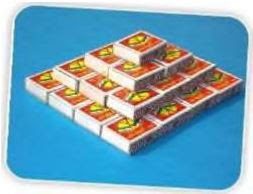• In the bottom layer, we can see that there are 4 matchboxes in length and 4 in breadth. So, the bottom layer has 16 matchboxes.

In the layer above the bottom, we can see that there are 3 matchboxes in length and 3 in breadth. So, the layer above bottom has 9 matchboxes.

Now, the second layer from the top has 2 matchboxes in length and 2 matchboxes in breadth. So, this layer has 4 matchboxes.

The top layer has only I matchbox. Thus, total volume of the arrangement in terms of matchboxes = 16 + 9 + 4 + 1= 30

Disclaimer: The answer may vary from student to student, based on his/her observation. It is highly recommended that the student prepare the answer on their own.

Page No 194:

Question 1:

(a) How long is the side of your cube? ____

(b) How many centimetre cubes can be arranged along its: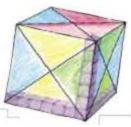• Length? _____
• Width? _____
• Height? _____To make the first layer on the table how many cm cubes will I use? How many such layers will I need to make? (d) So the total cm cubes = _____ (e) The volume of the paper cube is same as _____ cm cubes.

(a) Length of the side of the cube = 7 cm

(b) Number of centimeter cubes that can be arranged along its:

• Length? 7
• Width? 7
• Height? 7

(c) Answer Thimpu's questions: To make the first layer on the table, I use 49 cubes. I need 7 such layers to make the cube.

(d) So the total cm cubes = 7 x 7 x7 = 343

(e) The volume of the paper cube is the same as 343 cm cubes.

Page No 195:

Question 1: Anan made a big cube having double the side of your paper cube. How many of your paper cubes will fit in it? Try doing it by collecting all the cubes made in your class.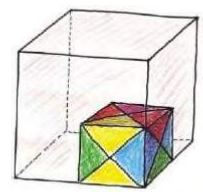Length of the side of paper cube = 7 cm Length of the side of Anan's cube =2 x 7 cm = 14 cm

We can fit 4 paper cubes, each of side 7 cm in the first layer of the big cube. As the length of each side of the big cube is 14 cm, there will be a total of 2 layers with each layer containing 4 paper cubes.

So, number of paper cubes in 2 layers = 2 × 4 = 8

Thus, 8 paper cubes will fit inside the Anan's big cube.

Question 2: Ganesh and Dinga want to pack 4000 centimetre cubes in boxes. These are to be sent to a school. There are three different boxes available for packing.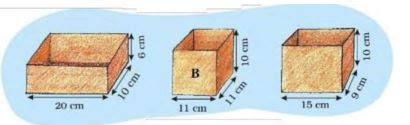• What is your guess? Who is right?
• How can Ganesh and Dinga test their guesses before packing the cubes in the boxes? Discuss with your friend. Use Ganesh's method and write:
• _____ centimetre cubes can be arranged in box B.
• _____ centimetre cubes can be arranged in box C.
• So_____ centimetre cubes in all can be packed in the three boxes.

• I guess that the cubes will fit in all the 3 boxes put together. Dinga's guess is correct.

• First of all, we will find how many cubes each box will contain, and then all the cubes will be added together to cross-check the guess.

• In the first layer of box B, we can keep 11 x 11 = 121 cubes. There are 10 such layers. So, in box B, we can arrange 10 x 121 = 1210 cubes

• In the first layer of box C, we can keep 15 x 9= 135 cubes. There are 10 such layers. So, in box C, we can arrange 10 x 135 = 1350 cubes

• In all the three boxes we can arrange 1200 + 1210 + 1350 = 3760 cubes So, 3760 centimetre cubes in total can be packed in three boxes.

Disclaimer: The first two part of the answer may vary from student to student. The answers provided here are for reference only.

Page No 196:

Question 1: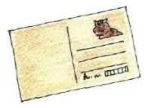Collect some old postcards. You can also use thick paper of size 14 cm x 9 cm. Fold the postcard along the width to make pipe-1. Join the ends with cello tape. Take another postcard and fold it along the length to make pipe-2. Join the ends with tape. Guess which pipe can take more sand inside it. Hold it on a plate and pour sand to check your guess. Was your guess correct? Discuss. Now do the same with other pipes shown here. To make the triangle-shaped pipe-3, draw two lines on the postcard. Fold the postcard along the lines. Join the ends with tape. Now make the square- shaped pipe-4. Find out which pipe can take the most sand inside it. So which pipe has the most volume?

Disclaimer: The answer may vary from student to student, based on his/her observation. It is highly recommended that the students prepare the answer on their own.

Page No 197:

Question 1: The students of Class XII are going on a trek to Gangotri. They have to pack their bags for six days and keep them light. They also have to take things that do not take too much space. So they will look for things that have both less volume and less weight. After all, they will carry their own bags while climbing the mountains! They even dry the onions and tomatoes to make them light. One kg of onions or tomatoes becomes 100 g when the water inside dries up. The list of food each person will need for one day:

• Rice: 100 g
• Flour (Atta): 100 g
• Pulses (Dal): 1/3 the weight of rice and flour
• Oil: 50 g
• Sugar: 50 g
• Milk powder: 40 g (for tea, porridge, and hot drink).
• Tea: Around 10 g
• Dalia: 40 g for breakfast.
• Salt: 5 g
• Dried onions: 10 g
• Dried tomatoes: 10 g

(a) For 6 days, each person will need

• Rice and flour- _____ g
• Pulses - _____ g
• Dried onions - _____ g

(b) How much of fresh tomatoes should be dried for 6 days for 10 people?

(c) What is the total weight of food (for 6 days) in each person's bag?

(a) Rice required per person per day = 100 g Flour required per person per day = 100 g Total rice                 and flour required for each person for a single day = 200 g Thus, for 6 days, rice and flour                       required per person = 200 g x 6 = 1200 g

• Pulses required per person per day = 1/3 the weight of rice and flour Pulses required per person for days 1200 g x 1/3 = 400 g
• Dried onions required per person per day = 10 g

For 6 days, dried onions required per person = 6 × 10 g = 60 g

(b) On drying 1 kg fresh tomatoes, we get 100 g of dried tomatoes. 1 kg = 1000 g It means for 100 g            dried tomatoes, we need 1000 g of fresh tomatoes.

So, for 1 g dried tomato, we need 1000/100 = 10g fresh tomatoes.

Hence, for 10 g dried tomatoes, we need 10 x 10 g = 100 g fresh tomatoes.

Thus, for 6 days, we need to dry 6 x 100 g = 600 g of fresh tomatoes.

(c) Total weight of food in each person's bag = 600 g + 600 g + 300 g + 50 g × 6 +50 g 6 +40 g 6                 +10 g x 6 + 40 g 6 + 5 g x 6 +10 g × 6 +10 g × 6 = 2790 g

Page No 199:

Question 1:

• Guess how many children of your weight will be equal to the weight of an elephant of 5000 kg.
• At birth, a baby elephant weighs around 90 kg. How much did you weigh when you were born? Find out. How many times is a baby elephant heavier than you were at birth?
• If a grown up elephant eats 136 kg of food in a day then it will eat around _____ kg in a month. Guess about how much it will eat in a year.

• Weight of a child of my age = 30 kg Weight of an elephant = 5000 kg Total number of children weighing 5000 kg = 5000/30 = 167 (approximately)
• Weight of a baby elephant = 90 kg My weight at birth = 3 kg Number of times a baby elephant was heavier than me at birth = 90/30 =30 So, the baby elephant was 30 times heavier than me at birth.
• Food eaten by a grown up elephant in 1 day = 136 kg Food eaten by a grown up elephant in 30 days = 30 x 136 = 4080 kg

In a year, it will eat around 50,000 kg of food.

Page No 200:

Question 1:

.How many coins are there in a sack of 5 rupee coins if it weighs:

(a) 18 kg?____(b) 54 kg?____ (c) 4500 g?____ (d) 2 kg ____

and 250 g? ____ (e) 1 kg and 125 g? ____

.A 2-rupee coin weighs 6 g. What is the weight of a sack with?

(a) 2200 coins? ____ kg ____ g (b) 3000 coins? ____ kg.

.If 100 one rupee coins weight 485 g then how much will 10000 coins' weight?____ kg ­____ g

1 kg = 1000 g Weight of a 5-rupee coin = 9 g

(a) Weight of 18 kg sack in grams = 18 x 1000 = 18000 g Number of 5 rupee coins in 18 kg sack = 18000 ÷ 9 = 2000

(b) Weight of 54 kg of sack in grams = 54 x 1000 = 54000 g Number of 5 rupee coins in 54 kg sack = 54000 ÷ 9 = 6000

(c) Weight of sack = 4500 g Number of 5 rupee coins in 4500 g sack = 4500 ÷ 9 = 500

(d) Weight of 2 kg 250 g sack = 2 × 1000 g + 250 g = 2000 g +250 g = 2250 g Number of 5 rupee coins in 2250 g sack = 2250 ÷ 9 = 250

(e) Weight of 1 kg 125 g sack = 1 x 1000 g + 125 g = 1000 g + 125 g = 1125 g

Number of 5 rupee coins in 1125 g sack = 1125  ÷ 9 = 125

.We know, 1 kg = 1000 g Weight of a 2-rupee coin = 6 g

(a) Now, weight of sack with 2200 coins = 2200 x 6 =13200 g = 13 × 1000 g +200 g = 13 kg 200 g

(b) Weight of the sack with 3000 coins = 3000 × 6=18000 g Thus, 18000 g= 18 x 1000 g = 18 kg

.Weight of 100 one-rupee coins = 485 g So, weight of a single one-rupee = 485/100 = 4.85 g Thus, weight of 10000 one-rupee coins = 10000 × 4.85= 48500 g

So, 48500 g = 48 x 1000 g + 500 g = 48 kg 500 g

Question 2:

• How do people who cannot see make out different notes and coins?
• What should we look for to check if a 100-rupee note is real or fake?